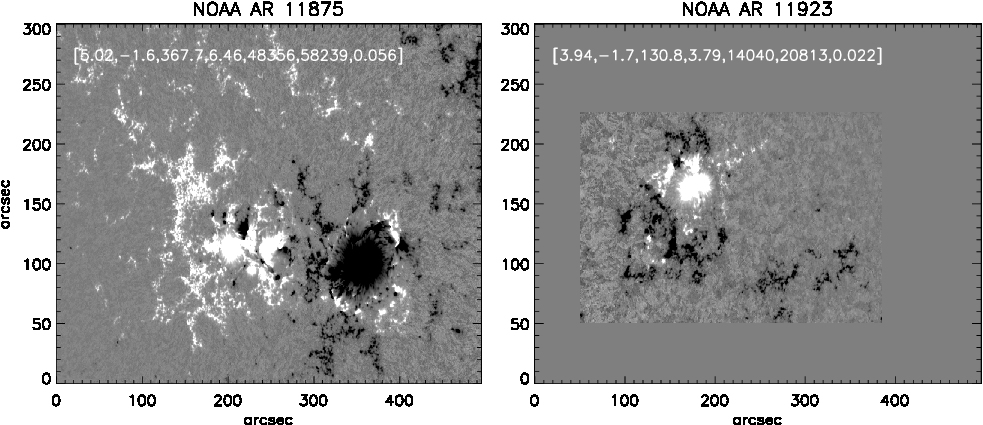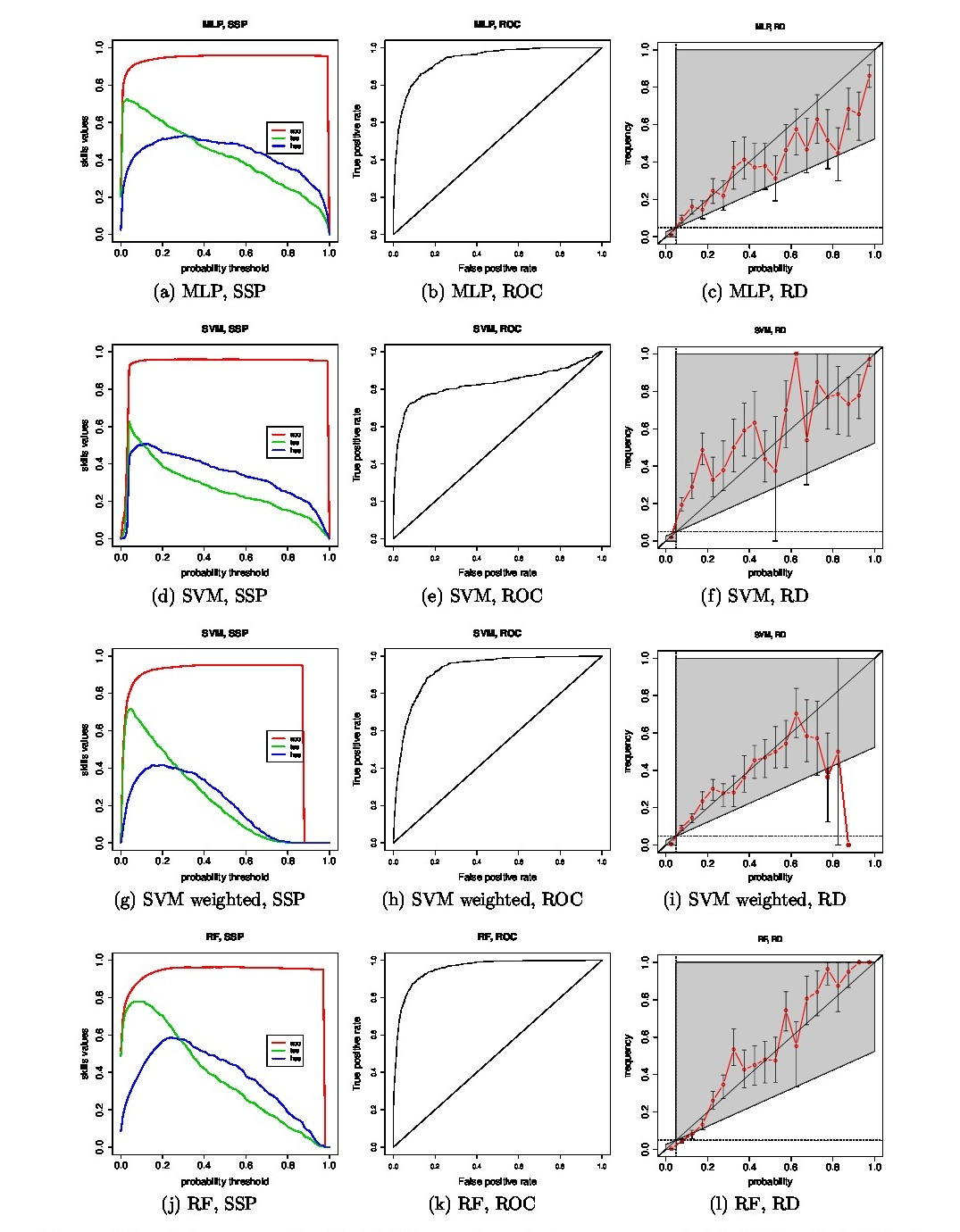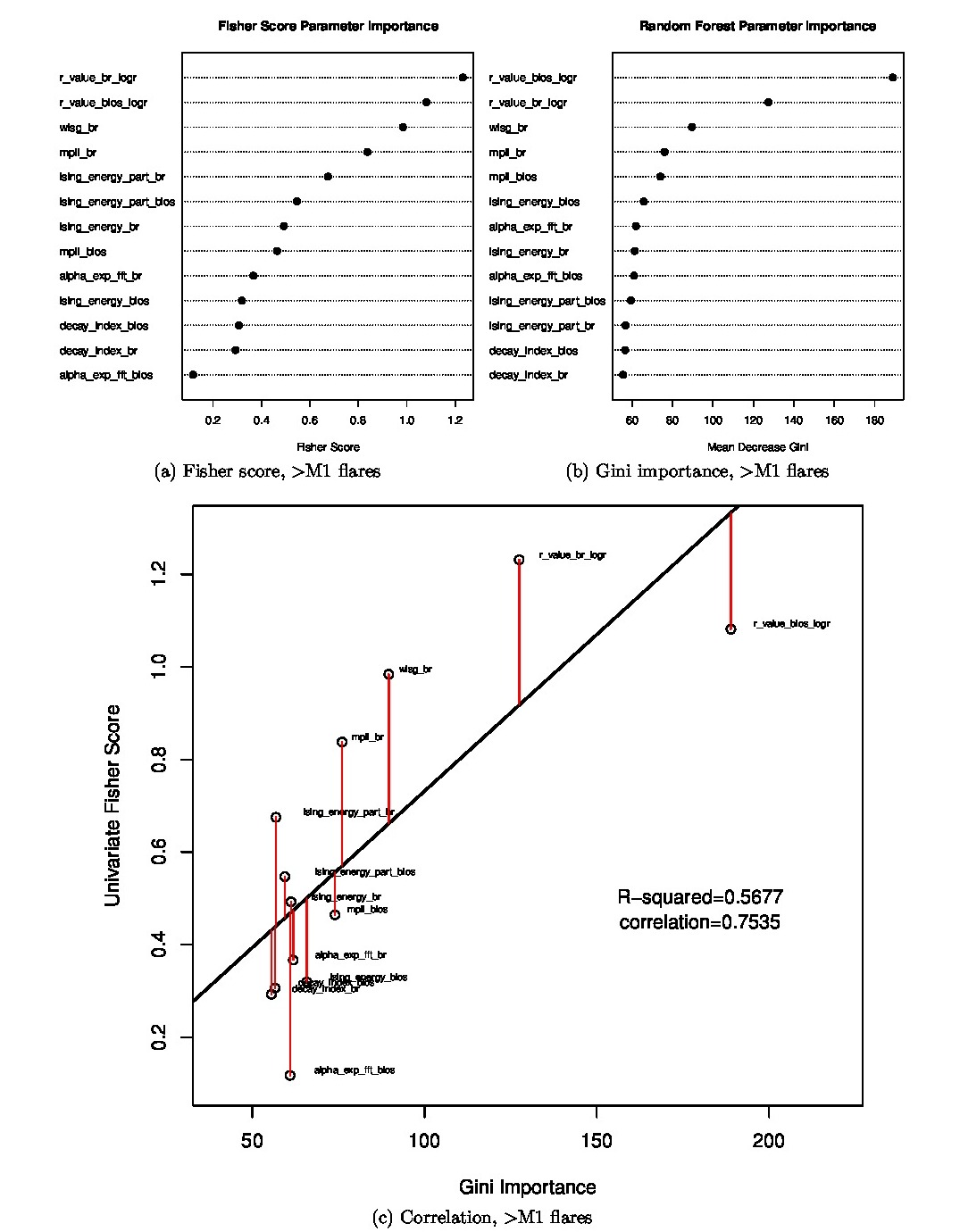# 95. Forecasting Solar Flares Using Magnetogram-Based Predictors and Machine Learning

Contributed by Kostas Florios. Posted on April 16, 2018

Kostas Florios1,2, Ioannis Kontogiannis1, Sung-Hong Park3, Jordan A. Guerra3, Federico Benvenuto4, D. Shaun Bloomfield5, Manolis K. Georgoulis1

2. Athens University of Economics and Business, Greece
3. School of Physics, Trinity College Dublin, Ireland
4. Dipartimento di Matematica, Universitàdi Genova, Italy
5. Northumbria University, Newcastle upon Tyne, NE1 8ST, UK

##### Abstract

A set of parameters that characterize the complexity and energy potential of solar active-regions is fed through several Machine Learning (ML) and conventional statistics algorithms to forecast solar flares. Our aim is to evaluate both algorithms and predictors. A statistically significant sample of 23,134 Space-weather HMI Active Region Patches (SHARPs) taken between 2012 – 2016 was used. We assess the quality of probabilistic forecasts using relevant metrics. The importance of the numerous magnetic predictors is ranked and partial models (e.g., involving subsets of the predictors) are constructed and put to work. Also, an operationally realistic scenario of “one forecast issued per day” is evaluated in contrast to the 8 forecasts per day baseline scenario. A Monte Carlo simulation showed that the Random Forest method provides an accuracy of ACC=0.93(0.00), true skill statistic TSS=0.74(0.02) and Heidke skill score HSS=0.49(0.01) for >M1 flare prediction with probability threshold of 15% and ACC=0.84(0.00), TSS=0.60(0.01), and HSS=0.59(0.01) for >C1 flare prediction with probability threshold 35%.

##### IntroductionFigure 1| Left: NOAA AR 11875, which produced 7 C-class flares within 24 h from observation. Right: NOAA AR 11923, which produced no flares. The two AR are plotted in a common frame of reference, to retain their original relative size. For comparison, vectors of the seven predictor values are included, [r_value_blos_logr, alpha_exp_fft_blos, mpil_blos, decay_index_blos, wlsg_blos, ising_energy_blos, ising_energy_part_blos]. High values of the predictors statistically indicate a powerful, potentially flare-prolific AR (left), with low values indicating a quiescent, flare-quiet AR (right).

We present an analysis regarding solar flare prediction based on SHARP magnetograms. We constructed an array of several quantities to be used as predictors of solar flare activity, namely Schrijver’s R, Fourier spectral power index, the Total Length of the Magnetic polarity inversion line, Decay index, Gradient-weighted integral length of the neutral line, and two versions of the Ising energy, of the original and the partitioned magnetograms[2,3]. Each of these components were calculated using both the line-of-sight (Blos) and the radial component of the magnetic field vector Br, thus comprising a set of fourteen predictors. For numerical reasons (e.g., presence of a few outlier observations) we did not use the gradient-weighted integral length of the neutral line in the line-of-sight reference system (wlsg_blos), so we ended with a set of K=13 predictors. The sampling period contained all the days from October 1, 2012 to January 13, 2016 at an intraday cadence of 3 h, starting at 00:00 UT. All SHARP cut-outs associated with a NOAA AR number were examined, leading to a sample size of 23,134 frames for each predictor. Figure 1 shows the values of seven predictors for two very different SHARP frames.

##### ResultFigure 2| ML methods comparison for >M1 GOES flares prediction for (from top to bottom) MLP, SVM, weighted SVM and RF. From left to right we present the corresponding SSP, ROC and RD.

We split the 23,134 observations in half, creating training and test sets. For a random split, Figure 2 shows the prediction performance of ML algorithms for >M1 flares. The ML algorithms, namely Random Forests (RF), Multi-Layer Perceptrons (MLP) and Support Vector Machines (SVM), provide a probability for the flare event (e.g., probabilistic classification). Thus, in Figure 2 the Skill Scores Profile (SSP), the Receiver Operating Characateristic (ROC) curve, and the Reliability Diagram (RD) are plotted as functions of the probability. The RF method has the better RD and SSP, as well as the largest area under the ROC among the ML methods for the >M1 flare prediction.Figure 3| Importance of several predictors while predicting >M1 flares.

Additional analysis for the >M1 flares shows the relative importance of every predictor in forecasting. Figure 3 reveals that Schrijver’s R, Gradient-weighted integral length of the neutral line, and Total Length of the Magnetic polarity inversion line are the most important predictors for >M1 flare forecasting in our sample. For more results, including results on the >C1 flare prediction, the reader is referred to our original publication, Ref 3.

### References

 Bobra, M., Sun, X., Hoeksema, J. T., et al. 2014, Solar Phys., 289, 3549
 Guerra, J. A., Park, S.-H., Gallagher, P. T., et al. 2018, Solar Phys., 293, 9
 Florios, K., Kontogiannis, I., Park, S.-H., et al. 2018, Solar Phys., 293, 28
 Brieman, L. 2001, Mach. Learn., 45, 5
 Chang, C.-C., & Lin, C.-J. 2011, ACM T. Intel. Syst. Tec., 2>, 27:1